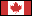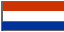## Search This Site## Browse by Vehicle Make and Model

All Makes & Models## Browse All Parts

Electric Scooter Repair Help > Modification and Custom Project Help > Bolt Circle Diameter Calculator

# Bolt Circle Diameter CalculatorBolt Circle Diameter Calculator

* Enter Parameters Below

 * Number of Bolt Holes: * Adjacent Bolt Hole Distance: Inches Millimeters Distance Type: Center To Center Back To Back

Bolt Circle Diameter Calculation Results
 Bolt Circle Diameter: Inches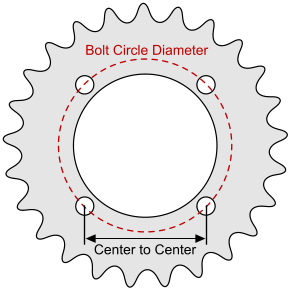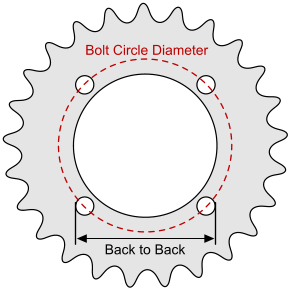This calculator provides a bolt circle diameter (BCD) measurement from the distance between two adjacent bolt holes. The bolt holes can be measured center to center or back to back so long as the the corresponding distance type is selected on the calculator. This calculator is most useful for sprockets with an odd number of bolt holes or for sprockets with an even number of bolt holes that are mounted on a wheel where there is an axle or rim hub that gets in the way of measuring the sprocket's bolt circle diameter.

# How To Measure Adjacent Bolt Hole Center To Center Distance

Step 1 - Measure Bolt Hole ID and Zero the Digital Caliper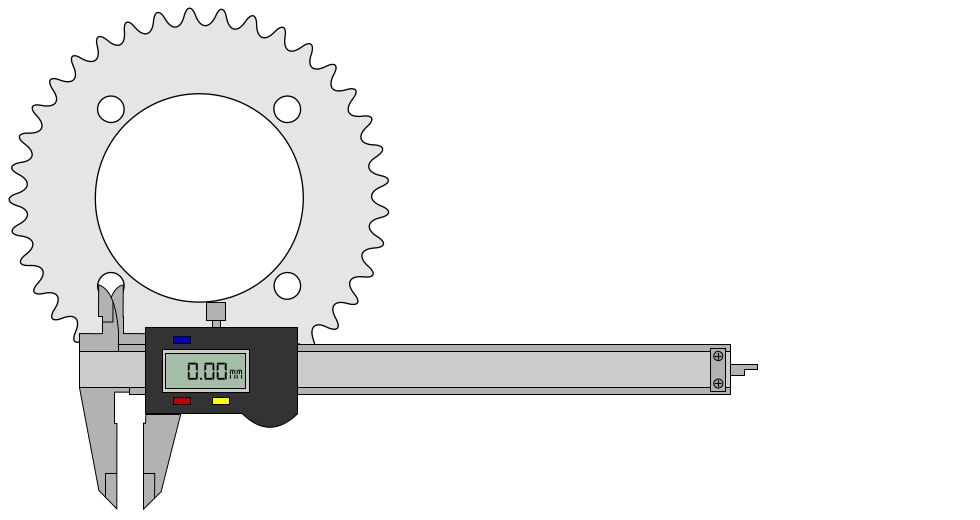Step 2 - Measure Adjacent Bolt Hole Back To Back Distance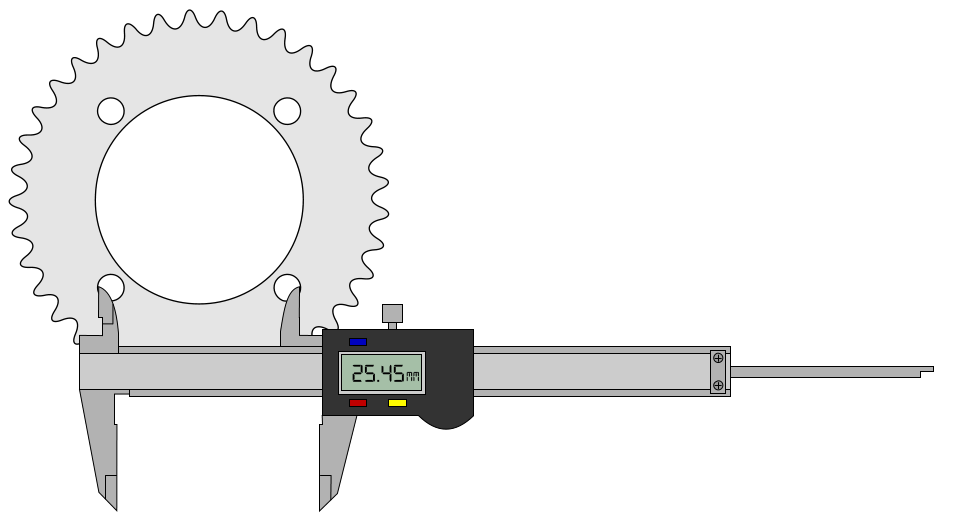Step 3 - The Digital Caliper Readout Is Now the Adjacent Bolt Hole Center To Center Distance

Measuring the adjacent bolt hole center to center distance or back to back distance and using the bolt circle diameter calculator located above or using a mathematical formula is the only way to determine the bolt circle diameter of sprockets, rims, axle hubs, and brake rotors with an odd number of bolt holes or with an even number of bolt holes where there is an axle or rim that gets in the way of measuring the diametrically opposed bolt holes to determine the bolt circle diameter.

# How To Measure Bolt Circle Diameter

Step 1 - Measure Bolt Hole ID and Zero the Digital CaliperStep 2 - Measure Diametrically Opposed Bolt Hole Back To Back Distance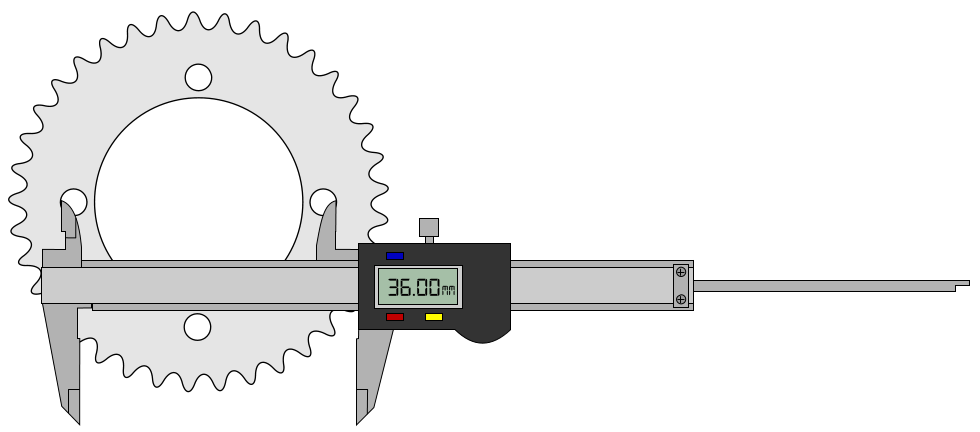Step 3 - The Digital Caliper Readout Is Now the Bolt Circle Diameter

Only the bolt circle diameter (BCD) of an even number of bolt holes can be measured this way. For sprockets, rims, axle hubs, and brake rotors with an odd number of bolt holes or with an even number of bolt holes where there is an axle or rim that gets in the way of measuring the diametrically opposed bolt holes the adjacent bolt hole distance will need to be measured and the bolt circle diameter calculator located above used to determine the bolt circle diameter.

## 1-800-908-8082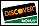## "When you need Electric Scooter Parts, go to: ElectricScooterParts.com"

We Ship Internationally